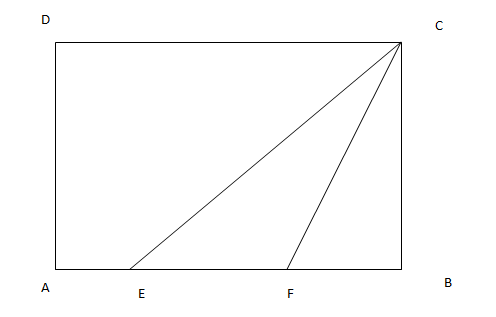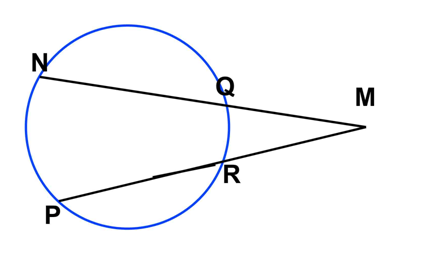## Geometry Test 1

Instructions

For the following questions answer them individually

Q 1

What is the area of a square of side 45 cm?

Q 2

An arc AB of a circle subtends an angle x radian at centre O of the circle. If the area of the sector AOB is equal to the square of the length of the arc AB, then x is:

Q 3

A rectangular pool of 20 m wide and 60 m long is surrounded by a walkway of uniform width. If the total area of the walkway is 516 $$m^2$$ , how wide, in metres, is the walkway?

Q 4

In the given diagram, ABCD is a rectangle with AE = EF = FB. What is the ratio of the areas of CEF and that of the rectangle?Q 5

In the given figure MN=14 cm , NQ = 3 cm, PM= 21cm. Find RM?